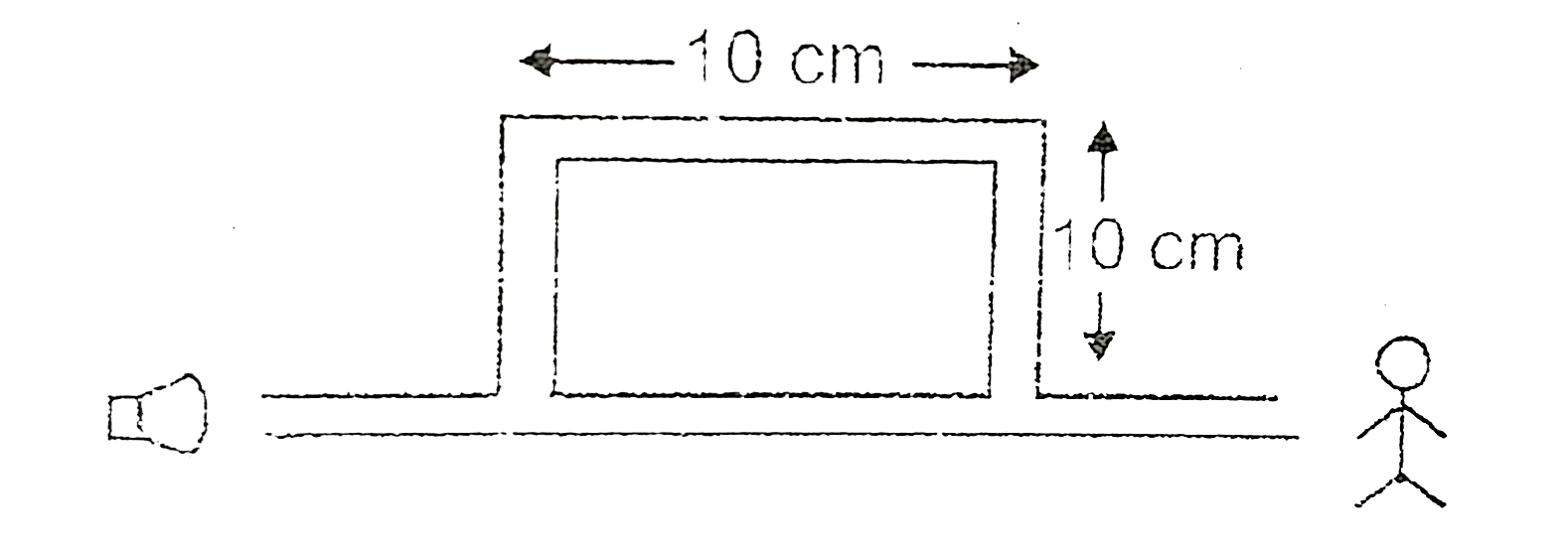# Figure shows a tube having sound source at one end and observe at other end. Source produces frequencies upto 10000 Hz. Speed of sound is 400 m//s

123 views
in Physics
closed
Figure shows a tube having sound source at one end and observe at other end. Source produces frequencies upto 10000 Hz. Speed of sound is 400 m//s. Find the frequencies at which person hears maximum intensity.by (85.2k points)
selected

The sound wave bifurcates at the juction of the straight and the rectangular parts. The wave through the straight part travels a distance p_(1) = 10 cm  and the wave through the rectangular part travels a distance p_(2) = 3 xx 10 xm = 30 before they mwwt agian and travel to the receiver. The path difference between the two wave received, therefore.
Deltap = p_(2) - p_(1) = 30 cm - 10 cm = 20 cm
The wavelength of either wave is (v)/(upsilon) rArr (400 m//s)/(upsilon). For constructive interference, Deltap = nlambda, where n is an integer.
or, Deltap = n.(v)/(v) rArr v = (n.v)/(Deltap)
rArr v = (400)/(0.1) = 4000 n
Thus, the frequencies within the specified range which cause maximum of intensity are
4000 xx 1 Hz, 4000 xx 2 Hz